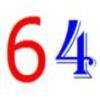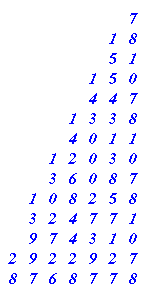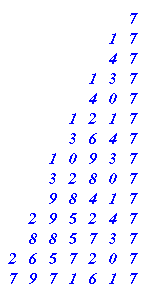#### You may also like### Exploring Wild & Wonderful Number Patterns

EWWNP means Exploring Wild and Wonderful Number Patterns Created by Yourself! Investigate what happens if we create number patterns using some simple rules.Write the numbers up to 64 in an interesting way so that the shape they make at the end is interesting, different, more exciting ... than just a square.Make a set of numbers that use all the digits from 1 to 9, once and once only. Add them up. The result is divisible by 9. Add each of the digits in the new number. What is their sum? Now try some other possibilities for yourself!

# Number Pattern Explorations

##### Age 7 to 14Challenge Level
You've probably come across number patterns before; ones like :-

2 4 6 8 10 12 . . .

512 256 128 64 32 . . .

220 210 200 190 180 170 . . .

11 14 17 20 23 26 . . .

Work out the rules that produced each of the patterns.

What could be the reason for the series of dots appearing after each one?

Well, let's form some patterns of our own. But this time we will use two maths operations [for the maths person 'operations' mean adding, multiplying, dividing, subtracting etc.] to produce each new number in the pattern. You can probably multiply, add, subtract and divide. Because dividing sometimes can leave bits left over, let's ignore that one for the time being.

Adding put together with subtracting results in something that we could have got just by adding or subtracting. Let me show you:-

Suppose you decided that you would add 5 and subtract 2 each time . . . . then you might as well just be adding 3 each time.

OR suppose you decided to add 6 and subtract 11 each time . . . . then you might as well just be taking away 5 each time.

I therefore suggest that we make a rule about the two operations that we will use for this exploration.

RULE 1 You will use multiplication with either adding or subtraction. BUT you can have the multiplication last if you like.

So let's get started, you will need to do 5 things for this work:

a) You need to choose a STARTING NUMBER (In my example suppose it's 7)
b) You need to decide which OPERATION to do first [addition, subtraction or multiplication] (In my example suppose it's multiplication )
c) You need to decide what NUMBER to use with the operation you chose in b (In my example suppose it's 3 )
d) You need to decide on your next OPERATION (I chose to use multiplication before so it's got to be addition or subtraction, suppose it's subtraction)
e) You need to decide what NUMBER to use with the operation you chose in d. (In my example suppose it's 3)

Let's run through this a bit.

Starting number 7 [ x 3 - 3 ] gives 18

Now we use 18..... [ x 3 - 3 ] gives 51

Now we use 51..... [ x 3 - 3 ] gives 150

I think it would be better to write it down without the brackets bit, although we do have to do both operations before we write the result down and it would be helpful to write it all out in columns going down:-Things like:-

The answers go odd, even, odd, even . . . . .

The units figures go 7 8 1 0 , 7 8 1 0 , . . . etc.

You might be able to see lots more, I've just written down a few quick ones.

When I've had a good long, hard look and talked with others, perhaps, I do the next stage; which is:-

Take a look at a, b, c, d, e, that I used and make a SMALL CHANGE

SUPPOSE I decide to change e, to subtracting 4 instead of 3 BUT everything else stays the same so:-

Starting Number 7 [ x 3 - 4 ] gives ......Like before I write down all the things that I notice :-

LIKE :-

The answers all end in a 7

The tens figures go 4 3 0 1, 4 3 0 1 . . .

AND maybe many more things.

Then I COMPARE what I noticed this time with last time, similar things and rather different things.

SO I might write something like:-

In the first pattern the units went in a pattern of 4 repeating different figures, in the second pattern it's the tens that do that. Both patterns end with a 7 every 4th one. I also notice that in the units of the first you could say that the 8 - 1 gives 7!

WELL after that L O N G introduction it's time for you to have a go!

1) Choose you five things to start off with a, b, c, d, e.
2) Produce at least 8 answers underneath each other in good columns [it helps to see tens patterns etc.].
3) Write about the things you notice.
4) Make one small change in the 5 starting things a, b, c, d, e.
5) Do 2) and 3) again.
6) Compare what's happened this time with the first time.

Then CHOOSE :-

i) It might be good to make a similar change again and see what happens [like subtracting 5 in our example]
ii) Make a very different change [so it might be the starting number this time changed to 10 in our example]
iii) Make a totally fresh new start [for example 3 ( x 11 + 1)]
iv) Follow something through that it interesting you when you compare what you notice.

If you notice something very interesting happen then you may be able to do some predicting and then asking yourself WHY does this particular thing happen.